# The CALIS Procedure

### The PATH Model

Subsections:

The PATH modeling language is supported in PROC CALIS as a more intuitive modeling tool. It is designed so that specification by using the PATH modeling language translates effortlessly from the path diagram. For example, consider the following simple path diagram:

Figure 29.3: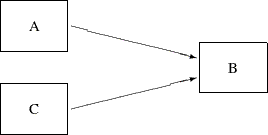You can use the following PATH statement to specify the paths easily:

```path    A ===> B ,
C ===> B ;
```

There are two path entries in the PATH statement: one is for the path `A ===> B`, and the other is for the path `C ===> B`. Sometimes you might want to name the effect parameters in the path diagram, as shown in the following:

Figure 29.4: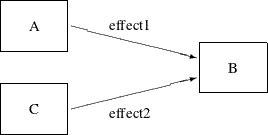You can specify the paths and the parameters together in the following statement:

```path    A ===> B   = effect1,
C ===> B   = effect2;
```

In the first entry of the PATH statement, the path `A ===> B` is specified together with the path coefficient (effect) `effect1`. Similarly, in the second entry, the `C ===> B` path is specified together with the effect parameter `effect2`. In addition to the path coefficients (effects) in the path diagram, you can also specify other types of parameters by using the PVAR and PCOV statements. See the section A Structural Equation Example for a more detailed example of the PATH model specification.

Despite its simple representation of the path diagram, the PATH modeling language is general enough to handle a wide class of structural models that can also be handled by other general modeling languages such as LINEQS, LISMOD, or RAM. For brevity, models specified by the PATH modeling language are called PATH models.

#### Types of Variables in the PATH Model

When you specify the paths in the PATH model, you typically use arrows (such as `<===` or `===>`) to denote "causal" paths. For example, in the preceding path diagram or the PATH statement, you specify that `B` is an outcome variable with predictors `A` and `C`, respectively, in two paths. An outcome variable is the variable being pointed to in a path specification, while the predictor variable is the one where the arrow starts from.

Whereas the outcome–predictor relationship describes the roles of variables in each single path, the endogenous–exogenous relationship describes the roles of variables in the entire system of paths. In a system of path specification, a variable is endogenous if it is pointed to by at least one single-headed arrow or it serves as an outcome variable in at least one path. Otherwise, it is exogenous. In the preceding path diagram, for example, variable `B` is endogenous and both variables `A` and `C` are exogenous. Note that although any variable that serves as an outcome variable at least in one path must be endogenous, it does not mean that all endogenous variables must serve only as outcome variables in all paths. An endogenous variable in a model might also serve as a predictor variable in a path. For example, variable `B` in the following PATH statement is an endogenous variable, and it serves as an outcome variable in the first path but as a predictor variable in the second path.

```path    A ===> B   = effect1,
B ===> C   = effect2;
```

A variable is a manifest or observed variable in the PATH model if it is measured and exists in the input data set. Otherwise, it is a latent variable. Because error variables are not explicitly defined in the PATH modeling language, all latent variables that are named in the PATH model are factors, which are considered to be the systematic source of effects in the model. Each manifest variable in the PATH model can be endogenous or exogenous. The same is true for any latent factor in the PATH model.

Because you do not name error variables in the PATH model, you do not need to specify paths from errors to any endogenous variables. Error terms are implicitly assumed for all endogenous variables in the PATH model. Although error variables are not named in the PATH model, the error variances are expressed equivalently as partial variances of the associated endogenous variables. These partial variances are set by default in the PATH modeling language. Therefore, you do not need to specify error variance parameters explicitly unless constraints on these parameters are desirable in the model. You can use the PVAR statement to specify the error variance or partial variance parameters explicitly.

#### Naming Variables in the PATH Model

Manifest variables in the PATH model are referenced in the input data set. Their names must not be longer than 32 characters. There are no further restrictions beyond those required by the SAS System. You use the names of manifest variables directly in the PATH model specification.

Because you do not name error variables in the PATH model, all latent variables named in the PATH model specification are factors (non-errors). Factor names in the PATH model must not be longer than 32 characters, and they should be different from the manifest variables. Unlike the LINEQS model, you do not need to use 'F' or 'f' prefix to denote latent factors in the PATH model. As a general naming convention, you should not use `Intercept` as either a manifest or latent variable name. See the section Naming Variables and Parameters for these general rules about naming variables and parameters.

#### Specification of the PATH Model

##### (1) Specification of Effects or Paths

You specify the "causal" paths or linear functional relationships among variables in the PATH statement. For example, if there is a path from `v2` to `v1` in your model and the effect parameter is named `parm1` with a starting value at 0.5, you can use either of these specifications:

```path     v1 <===  v2    = parm1(0.5);
```
```path     v2 ===>  v1    = parm1(0.5);
```

If you have more than one path in your model, path specifications should be separated by commas, as shown in the following PATH statement:

```path
v1 <===  v2   = parm1(0.5),
v2 <===  v3   = parm2(0.3);
```

Because the PATH statement can be used only once in each model specification, all paths in the model must be specified together in a single PATH statement. See the for more details about the syntax.

##### (2) Specification of Variances and Partial (Error) Variances

If `v2` is an exogenous variable in the PATH model and you want to specify its variance as a parameter named `parm2` with a starting value at 10, you can use the following PVAR statement specification:

```pvar     v2  = parm2(10.);
```

If `v1` is an endogenous variable in the PATH model and you want to specify its partial variance or error variance as a parameter named `parm3` with a starting value at 5.0, you can also use the following PVAR statement specification:

```pvar     v1 = parm3(5.0);
```

Therefore, the PVAR statement can be used for both exogenous and endogenous variables. When a variable in the statement is exogenous (which can be automatically determined by PROC CALIS), you are specifying the variance parameter of the variable. Otherwise, you are specifying the partial or error variance for an endogenous variable.

You do not need to supply the parameter names for the variances or partial variances if these parameters are not constrained. For example, the following statement specifies the unnamed free parameters for variances or partial variances of `v1` and `v2`:

```pvar     v1 v2;
```

If you have more than one variance or partial variance parameter to specify in your model, you can put a variable list on the left-hand side of the equal sign, and a parameter list on the right-hand side, as shown in the following PVAR statement specification:

```pvar
v1 v2 v3 = parm1(0.5) parm2 parm3;
```

In the specification, variance or partial variance parameters for variables `v1``v3` are `parm1`, `parm2`, and `parm3`, respectively. Only `parm1` is given an initial value at 0.5. The initial values for other parameters are generated by PROC CALIS.

You can also separate the specifications into several entries in the PVAR statement. Entries should be separated by commas. For example, the preceding specification is equivalent to the following specification:

```pvar
v1 = parm1 (0.5),
v2 = parm2,
v3 = parm3;
```

Because the PVAR statement can be used only once in each model specification, all variance and partial variance parameters in the model must be specified together in a single PVAR statement. See the for more details about the syntax.

##### (3) Specification of Covariances and Partial Covariances

If you want to specify the (partial) covariance between two variables `v3` and `v4` as a parameter named `parm4` with a starting value at 3, you can use the following PCOV statement specification:

```pcov  v3  v4 = parm4 (5.);
```

Whether `parm4` is a covariance or partial covariance parameter depends on the variable types of `v3` and `v4`. If both `v3` and `v4` are exogenous variables (manifest or latent), `parm4` is a covariance parameter between `v3` and `v4`. If both `v3` and `v4` are endogenous variables (manifest or latent), `parm4` is a parameter for the covariance between the errors for `v3` and `v4`. In other words, it is a partial covariance or error covariance parameter for `v3` and `v4`.

A less common case is when one of the variables is exogenous and the other is endogenous. In this case, `parm4` is a parameter for the partial covariance between the endogenous variable and the exogenous variable, or the covariance between the error for the endogenous variable and the exogenous variable. Fortunately, such covariances are relatively uncommon in statistical modeling. Their uses confuse the roles of systematic and unsystematic sources in the model and lead to difficulties in interpretations. Therefore, you should almost always avoid this kind of partial covariance.

Like the syntax of the PVAR statement, you can specify a list of (partial) covariance parameters in the PCOV statement. For example, consider the following statement:

```pcov
v1 v2 = parm4,
v1 v3 = parm5,
v2 v3 = parm6;
```

In the specification, three (partial) covariance parameters `parm4`, `parm5`, and `parm6` are specified, respectively, for the variable pairs (`v1`,`v2`), (`v1`,`v3`), and (`v2`,`v3`). Entries for (partial) covariance specification are separated by commas.

Again, if all these covariances are not constrained, you can omit the names for the parameters. For example, the preceding specification can be specified as the following statement when the three covariances are free parameters in the model:

```pcov
v1 v2,
v1 v3,
v2 v3;
```

Or, you can simply use the following within-list covariance specification:

```pcov
v1 v2 v3;
```

Three covariance parameters are generated by this specification.

Because the PCOV statement can be used only once in each model specification, all covariance and partial covariance parameters in the model must be specified together in a single PCOV statement. See the for more details about the syntax.

##### (4) Specification of Means and Intercepts

Means and intercepts are specified when the mean structures of the model are of interest. You can specify mean and intercept parameters in the MEAN statement. For example, consider the following statement:

```mean     V5 = parm5(11.);
```

If `V5` is an exogenous variable (which is determined by PROC CALIS automatically), you are specifying `parm5` as the mean parameter of `V5`. If `V5` is an endogenous variable, you are specifying `parm5` as the intercept parameter for `V5`.

Because each named variable in the PATH model is either exogenous or endogenous (exclusively), each variable in the PATH model would have either a mean or an intercept parameter (but not both) to specify in the MEAN statement. Like the syntax of the PVAR statement, you can specify a list of mean or intercept parameters in the MEAN statement. For example, in the following statement you specify a list of mean or intercept parameters for variables `v1``v4`:

```mean
v1-v4 = parm6-parm9;
```

This specification is equivalent to the following specification with four entries of parameter specifications:

```mean
v1 = parm6,
v2 = parm7,
v3 = parm8,
v4 = parm9;
```

Again, entries in the MEAN statement must be separated by commas, as shown in the preceding statement.

Because the MEAN statement can be used only once in each model specification, all mean and intercept parameters in the model must be specified together in a single MEAN statement. See the for more details about the syntax.

##### Specifying Parameters without Initial Values

If you do not have any knowledge about the initial value for a parameter, you can omit the initial value specification and let PROC CALIS compute it. For example, you can provide just the parameter locations and parameter names as in the following specification:

```path    v1 <=== v2   = parm1;
pvar v2 = parm2,
v1 = parm3;
```
##### Specifying Fixed Parameter Values

If you want to specify a fixed parameter value, you do not need to provide a parameter name. Instead, you provide the fixed value (without parentheses) in the specification.

For example, in the following statement the path coefficient for the path is fixed at 1.0 and the (partial) variance of `F1` is also fixed at 1.0:

```path    v1 <=== F1  = 1.;
pvar
F1 = 1.;
```
##### A Complete PATH Model Specification Example

The following specification shows a more complete PATH model specification:

```path     v1 <===  v2 ,
v1 <===  v3 ;
pvar  v1,
v2 = parm3,
v3 = parm3;
pcov  v3 v2 =  parm5(5.);
```

The two paths specified in the PATH statement have unnamed free effect parameters. These parameters are named by PROC CALIS with the `_Parm` prefix and unique integer suffixes. The error variance of `v1` is an unnamed parameter, while the variances of `v2` and `v3` are constrained by using the same parameter `parm3`. The covariance between `v2` and `v3` is a free parameter named `parm5`, with a starting value of 5.0.

#### Default Parameters in the PATH Model

There are two types of default parameters of the PATH model. One is the free parameters; the other is the fixed constants.

The following sets of parameters are free parameters by default:

• the variances or partial (or error) variances of all variables, manifest or latent

• the covariances among all exogenous (independent) manifest or latent variables

• the means of all exogenous (independent) manifest variables if the mean structures are modeled

• the intercepts of all endogenous (dependent) manifest variables if the mean structures are modeled

For each of the default free parameters, PROC CALIS generates a parameter name with the `_Add` prefix and a unique integer suffix. Parameters that are not default free parameters in the PATH model are fixed zeros by default. You can override almost all of the default zeros of the PATH model by using the MEAN, PATH, PCOV, and MEAN statements. The only exception is the single-headed path that has the same variable on both sides. That is, the following specification is not accepted by PROC CALIS:

```path     v1  <===  v1    = parm;
```

This path should always has a zero coefficient, which is treated as a model restriction that prevents a variable from having a direct effect on itself.

#### Relating the PATH Model to the RAM Model

Mathematically, the PATH model is essentially the RAM model. You can consider the PATH model to share exactly the same set of model matrices as in the RAM model. See the section Model Matrices in the RAM Model and the section Summary of Matrices and Submatrices in the RAM Model for details about the RAM model matrices. In the RAM model, the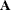matrix contains effects or path coefficients for describing relationships among variables. In the PATH model, you specify these effect or coefficient parameters in the PATH statement. The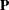matrix in the RAM model contains (partial) variance and (partial) covariance parameters. In the PATH model, you use the PVAR and PCOV statements to specify these parameters. The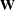vector in the RAM model contains the mean and intercept parameters, while in the PATH model you use the MEAN statement to specify these parameters. By using these model matrices in the PATH model, the covariance and mean structures are derived in the same way as they are derived in the RAM model. See the section The RAM Model for derivations of the model structures.